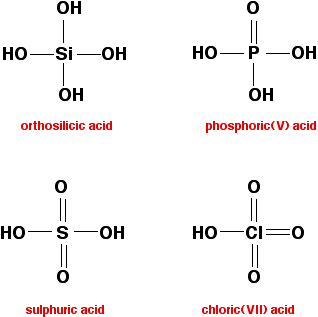# Hydroxides of Period 3 Elements

•• Contributed by Jim Clark
• Former Head of Chemistry and Head of Science at Truro School in Cornwall

This page briefly examines how the chemistry of the "hydroxides" of the Period 3 elements vary across the period, from sodium to chlorine.

## A quick summary of the trends

### Sodium and magnesium hydroxides

These contain hydroxide ions, and are simple basic hydroxides.

### Aluminum hydroxide

Aluminum hydroxide, like aluminum oxide, is amphoteric (it has both basic and acidic properties).

### The other "hydroxides"

All the other hydroxides have -OH groups covalently bound to the atom from period 3. These compounds are all acidic, ranging from the very weak silicic acids (one of which is shown below) to the very strong sulfuric or chloric(VII) acids.There are other acids (also containing -OH groups) formed by these elements, but these are the ones where the Period 3 element is in its highest oxidation state.

### Sodium and Magnesium Hydroxides

Sodium hydroxide and magnesium hydroxide are both basic because they contain hydroxide ions, which are strongly basic when solvated. Both species react with acids to form salts. For example, in dilute hydrochloric acid, sodium hydroxide and magnesium hydroxide form solutions of sodium chloride and magnesium chloride, respectively:

$NaOH + HCl \rightarrow NaCl + H_2O$

$Mg(OH)_2 + 2HCl \rightarrow MgCl_2 + 2H_2O$

### Aluminum Hydroxide

Aluminum hydroxide is amphoteric. Like sodium or magnesium hydroxides, it reacts with acids, showing basic character. When reacted with dilute hydrochloric acid, it forms a colorless solution of aluminum chloride:

$Al(OH)_3 + 3HCl \rightarrow AlC_3 +3H_2O$

Aluminum hydroxide can also act as an acid, reacting (for example) with sodium hydroxide to give a colorless solution of sodium tetrahydroxoaluminate:

$Al(OH)_3 + NaOH \rightarrow NaAl(OH)_4$

### The other "hydroxides"

None of these compounds contain hydroxide ions. In each case the -OH group is covalently bound to the Period 3 element, and in each case it is possible for the hydrogen atoms on these -OH groups to be removed by a base. In other words, these compounds are acidic. However, their acidities vary considerably in strength:

• Orthosilicic acid is very weak .
• Phosphoric(V) acid is weak, but somewhat stronger than simple organic acids like ethanoic acid.
• Sulfuric acid and chloric(VII) acid are both very strong.

The main consideration in determining the strength of the acid is the stability of the anion once the proton has been removed. This in turn depends on how much the negative charge can be delocalized (spread out) over the rest of the ion.

• If the negative charge is entirely on the oxygen atom left behind from the -OH group, the anion is very unstable and thus attractive to protons. The lost proton is easily recaptured; therefore, the acid is weak.
• Alternatively, if the charge can be distributed over the whole of the ion, the charge becomes so "dilute" that it cannot attract the hydrogen back very easily; therefore, the acid is strong.

In these compounds, the negative charge of the anion is delocalized through interaction with doubly-bonded oxygen atoms. For example, in chloric(VII) acid, the ion produced is the chlorate(VII) ion (also known as the perchlorate ion), ClO4-. The commonly-drawn structure of chloric acid, shown below, incorrectly shows the negative charge isolated to one oxygen atom:Instead, the charge is delocalized over the whole ion. Because of this, all four chlorine-oxygen bonds are identical, as shown:When sulfuric acid loses a hydrogen ion to form the hydrogen sulfate ion, HSO4-, the resulting negative charge is spread over three oxygen atoms (the original, deprotonated atom, and the two atoms doubly-bonded to sulfur). The charge is not as widely distributed as in perchlorate, but the delocalization is still quite effective; sulfuric acid is almost as strong as chloric(VII) acid.

Phosphoric(V) acid is much weaker than sulfuric acid because there is only one phosphorus-oxygen double bond which can be involved in charge delocalization; the charge is therefore delocalized less effectively.

In orthosilicic acid, there are no silicon-oxygen double bonds to delocalize the charge. The anion formed by deprotonation is not stabilized, and easily recovers its proton.

## Contributors

Jim Clark (Chemguide.co.uk)